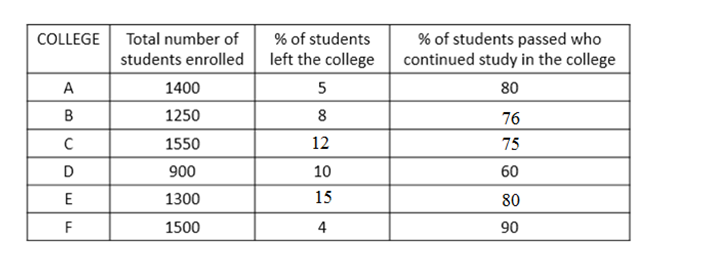# SBI Clerk Prelims 2021 Quantitative Aptitude Questions (Day-66)

Dear Aspirants, Our IBPS Guide team is providing new series of Quantitative Aptitude Questions for SBI Clerk Prelims 2020 so the aspirants can practice it on a daily basis. These questions are framed by our skilled experts after understanding your needs thoroughly. Aspirants can practice these new series questions daily to familiarize with the exact exam pattern and make your preparation effective.

Start Quiz

Missing number series

Directions (01-05): What value should come in the place of (?) in the following number series?

1) 13, 50, 135, 279, 426.5, ?

A.433.5

B.478.5

C.482.5

D.453.5

E.463.5

2) 8, 152, 273, ?, 454, 518

A.298

B.320

C.343

D.364

E.373

3) 19, 22, 30, 45, 69, ?

A.90

B.98

C.104

D.110

E.114

4) 23, 47, 75, 107, 143, ?

A.219

B.198

C.183

D.176

E.150

5) 19, 40, 84, 153, 249, ?

A.374

B.386

C.398

D.419

E.439

Data Interpretation

Directions (6-10): Study the following information carefully and answer the question given below.

The following table represents the number of students enrolled in six different colleges, percentage of students left the college and percentage of students who passed out the college among those who continued their study in the college.6) Find the sum of total number of students from all the colleges, who continued their study.

A.7432

B.7223

C.7199

D.7351

E.None of these

7) Number of students passed from college B is approximately what percent of the number of students passed from college D?

A.152

B.180

C.187

D.125

E.None of these

8) Total number of students enrolled in A and B together is what percent more than the number of students enrolled in C and D together?

A.6.134%

B.8.163%

C.9.156%

D.4.146%

178%

9) Find the ratio of number of failed students of college C and college F respectively.

A.1137 : 450

B.960 : 1457

C.341 : 144

D.450 : 137

E.None of these

10) Passed students of college A is approximately what percent of failed students of college C?

A.121 %

B.184 %

C.211 %

D.312 %

E.None of these

13 * 3 + 11 = 50

50 * 2.5 + 10 = 135

135 * 2 + 9 = 279

279 * 1.5 + 8 = 426.5

426.5 * 1 + 7 = 433.5

8 + 122 = 152

152 + 112 = 273

273 + 102 = 373

373 + 92 = 454

454 + 82 = 518

19 + (3 * 1) = 22

22 + (4 * 2) = 30

30 + (5 * 3) = 45

45 + (6 * 4) = 69

69 + (7 * 5) = 104

23 + 24 = 47

47 + 28 = 75

75 + 32 = 107

107 + 36 = 143

143 + 40 = 183

19 + (21 * 1) = 40

40 + (22 * 2) = 84

84 + (23 * 3) = 153

153 + (24 * 4) = 249

249 + (25 * 5) = 374

A = (100 – 5)/100 x 1400 = 1330

B = (100 – 8)/100 x 1250 = 1150

C = (100 – 12)/100 x 1550 = 1364

D = (100 – 10)/100 x 900 = 810

E = (100 – 15)/100 x 1300 = 1105

F = (100 – 4)/100 x 1500 = 1440

Required No of students = 1330 + 1150 + 1364 + 810 + 1105 + 1440 = 7199

Number of students who continued study in B = (100 – 8)/100 x 1250 = 1150

Passed Students = 76/100 x 1150 = 874

Number of students who continued study in D = (100 – 10)/100 x 900 = 810

Passed Students = 60/100 x 810 = 486

Required percentage = (874/486) x 100 = 179.83% = 180 %

Total number of students enrolled in A and B together = 1400 + 1250 = 2650

Total number of students enrolled in C and D together = 1550 + 900 = 2450

Required percentage = (2650 – 2450)/2450 x 100 = 8.163%

Number of students who continued study in C = (100 – 12)/100 x 1550 = 1364

Failed = (100-75)/100 x 1364 = 341

Number of students who continued study in F = (100 – 4)/100 x 1500 = 1440

Failed = (100 – 90)/100 x 1440 = 144

Required ratio = 341 : 144

Number of students who continued their study from college A = (100 – 5)/100 x 1400 = 1330

Passed = 80/100 x 1330 = 1064

Number of students who continued their study from college C

= (100 – 12)/100 x 1550 = 1364

Failed = (100 – 75)/100 x 1364 = 341

Required percentage = (1064/341) x 100 = 312.023% = 312 %

 Check Here to View SBI Clerk Prelims 2021 Quantitative Aptitude Questions Day – 65 Day – 64 Day – 63
2 1 vote
Rating1 Comment
Inline Feedbacks